# Imaginary unit facts for kids

Kids Encyclopedia Facts
(Redirected from Imaginary Unit)

In math, imaginary units, or$i$, are numbers that can be represented by equations but refer to values that could not physically exist in real life. The mathematical definition of an imaginary unit is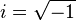$i = \sqrt{-1}$, which has the property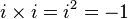$i \times i = i^2 = -1$.

The reason$i$ was created was to answer a polynomial equation,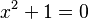$x^2 + 1 = 0$, which normally has no solution as the value of x^2 would have to equal -1. Though the problem is solvable, the square root of -1 could not be represented by a physical quantity of any objects in real life.

## Square root of i

It is sometimes assumed that one must create another number to show the square root of$i$, but that is not needed. The square root of$i$ can be written as: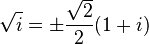$\sqrt{i} = \pm \frac{\sqrt{2}}{2} (1 + i)$.
This can be shown as: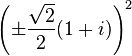$\left( \pm \frac{\sqrt{2}}{2} (1 + i) \right)^2 \$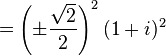$= \left( \pm \frac{\sqrt{2}}{2} \right)^2 (1 + i)^2 \$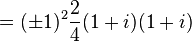$= (\pm 1)^2 \frac{2}{4} (1 + i)(1 + i) \$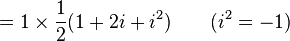$= 1 \times \frac{1}{2} (1 + 2i + i^2) \quad \quad (i^2 = -1) \$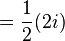$= \frac{1}{2} (2i) \$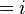$= i \$

## Powers of i

The powers of$i$ follow a predictable pattern: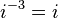$i^{-3} = i$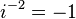$i^{-2} = -1$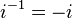$i^{-1} = -i$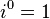$i^0 = 1$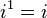$i^1 = i$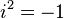$i^2 = -1$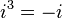$i^3 = -i$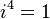$i^4 = 1$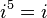$i^5 = i$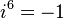$i^6 = -1$

This can be shown with the following pattern where n is any integer: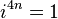$i^{4n} = 1$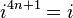$i^{4n+1} = i$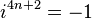$i^{4n+2} = -1$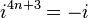$i^{4n+3} = -i$

## Related pagesImaginary unit Facts for Kids. Kiddle Encyclopedia.